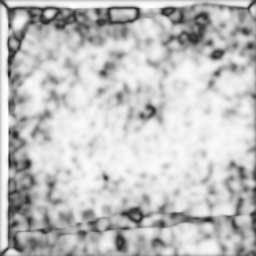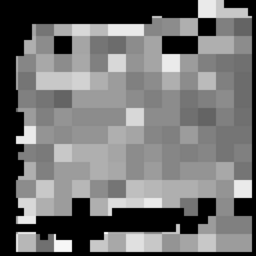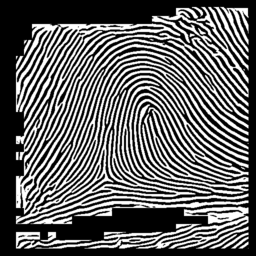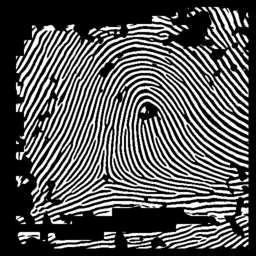## Example of fingerprint enhancement

This code loosely follows the approach presented by
Hong, L., Wan, Y., and Jain, A. K. 'Fingerprint image enhancement: Algorithm and performance evaluation'. IEEE Transactions on Pattern Analysis and Machine Intelligence 20, 8 (1998), pp 777-789.

Ridge regions in the image are identified and normalised, ridge orientations are determined, local ridge frequencies calculated, and then contextual filters with the appropriate orientation and frequency are applied.finger.png

```    im = imread('finger.png');

% Identify ridge-like regions and normalise image
blksze = 16; thresh = 0.1;
[normim, mask] = ridgesegment(im, blksze, thresh);
show(normim,1);

% Determine ridge orientations
[orientim, reliability] = ridgeorient(normim, 1, 5, 5);
plotridgeorient(orientim, 20, im, 2)
show(reliability,6)
```Orientations overlaid'Reliability' of orientations
```
% Determine ridge frequency values across the image
blksze = 36;
[freq, medfreq] = ridgefreq(normim, mask, orientim, blksze, 5, 5, 15);
show(freq,3)
```Frequency data

```
% Actually I find the median frequency value used across the whole
% fingerprint gives a more satisfactory result...

% Now apply filters to enhance the ridge pattern
newim = ridgefilter(normim, orientim, freq, 0.5, 0.5, 1);
show(newim,4);
```Filtered image

```
% Binarise, ridge/valley threshold is 0
binim = newim > 0;
show(binim,5);
```Binary image

```
% Display binary image for where the mask values are one and where
% the orientation reliability is greater than 0.5Masked binary imageOriginal image